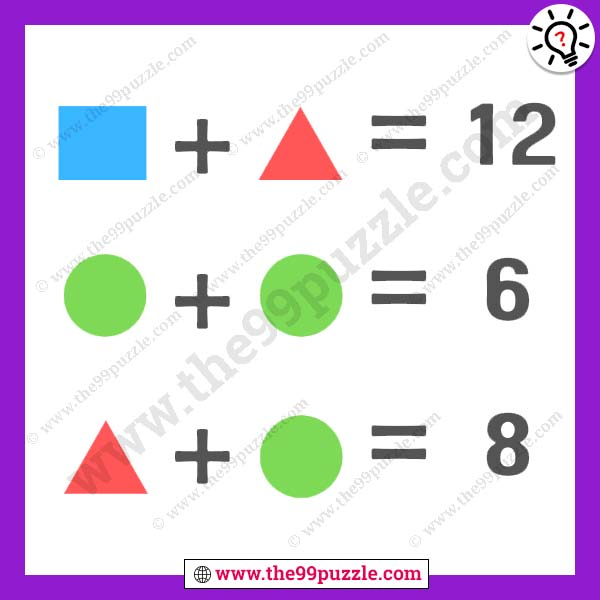# Find out the value of rectangle – Puzz269

This simple logic puzzle picture is for kids and adults. This math picture puzzle is viral on the internet. Here in this picture, you see three different shapes. Most people failed to answer these viral puzzles. If you understand the logic you can easily find out the value of rectangle. If you are a genius minded you can quickly solve picture puzzles.###### Explanation:

Rectangle+Triangle = 12

Circle+Circle = 6

Triangle+Circle = 8

7+5 = 12

3+3 = 6

5+3 = 8